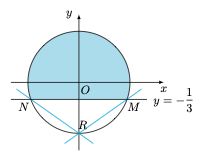# 每日一题椭圆的参数方程

1、求椭圆方程．

2、设直线 $PA$ 的斜率为 $k$，线段 $PB$ 的中垂线与 $y$ 轴交于点 $E$，若点 $E$ 在椭圆的外部，求斜率 $k$ 的取值范围．

1、$\dfrac{x^2}4+y^2=1$．

2、设 $B(2\cos\theta,\sin\theta)$，则线段 $PB$ 的中垂线$l:y=-\dfrac{2\cos\theta-0}{\sin\theta-1}(x-\cos\theta)+\dfrac{1+\sin\theta}2,$于是点 $E\left(0,-\dfrac 32(1+\sin\theta)\right)$．根据题意，有$\left|-\dfrac 32(1+\sin\theta)\right)>1\iff \sin\theta>-\dfrac 13.$因此直线 $PA$ 的斜率$k=\dfrac{1+\sin\theta}{2\cos\theta},$利用规划可得所求斜率 $k$ 的取值范围是 $\left(-\infty,-\dfrac{\sqrt 2}4\right)\cup\left(\dfrac{\sqrt 2}4,+\infty\right)$．